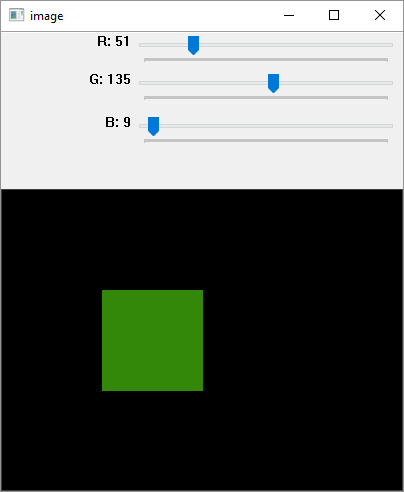# OpenCV Python - Add Trackbar

Trackbar in OpenCV is a slider control which helps in picking a value for the variable from a continuous range by manually sliding the tab over the bar. Position of the tab is synchronised with a value.

The createTrackbar() function creates a Trackbar object with the following command −

cv2.createTrackbar(trackbarname, winname, value, count, TrackbarCallback)


In the following example, three trackbars are provided for the user to set values of R, G and B from the grayscale range 0 to 255.

Using the track bar position values, a rectangle is drawn with the fill colour corresponding to RGB colour value.

## Example

Following program is for adding a trackbar −

import numpy as np
import cv2 as cv
img = np.zeros((300,400,3), np.uint8)
cv.namedWindow('image')
def nothing(x):
pass

# create trackbars for color change
cv.createTrackbar('R','image',0,255,nothing)
cv.createTrackbar('G','image',0,255,nothing)
cv.createTrackbar('B','image',0,255,nothing)

while(1):
cv.imshow('image',img)
k = cv.waitKey(1) & 0xFF
if k == 27:
break
# get current positions of four trackbars
r = cv.getTrackbarPos('R','image')
g = cv.getTrackbarPos('G','image')
b = cv.getTrackbarPos('B','image')

#s = cv.getTrackbarPos(switch,'image')
#img[:] = [b,g,r]
cv.rectangle(img, (100,100),(200,200), (b,g,r),-1)
cv.destroyAllWindows()


## Output